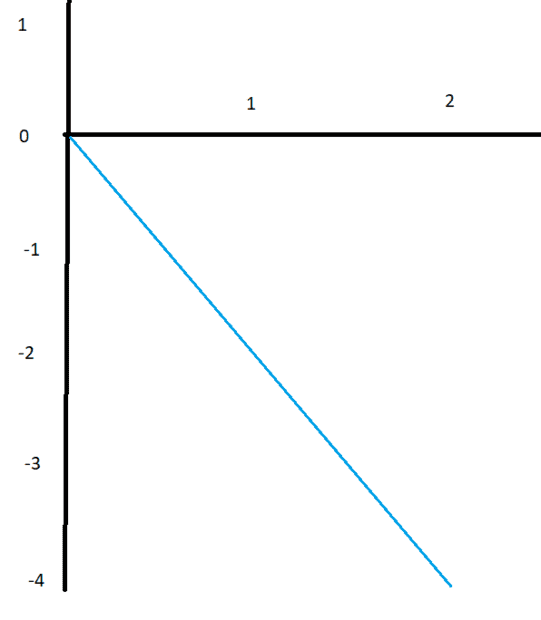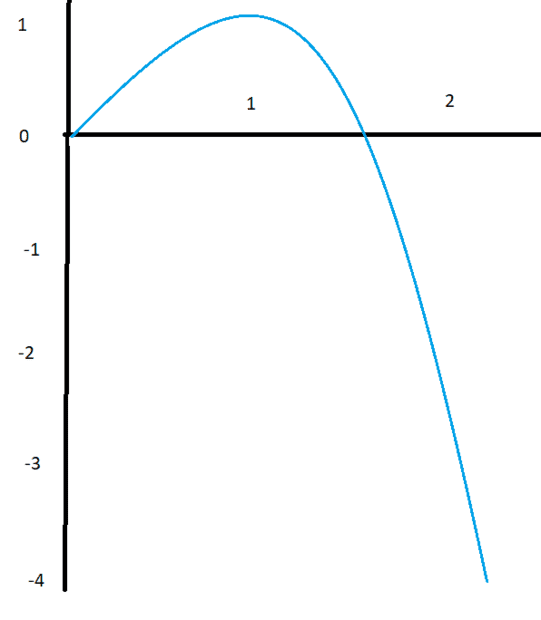# Motion along a straight line

Elementard
Homework Statement:
The velocity of an object is given as a function of time by v = 4t - 3t^2, where v is in m/s and t is in seconds. Its average velocity over the interval from t = 0 to t = 2s.
Relevant Equations:
i’m not sure. all I know is that the answer is 0m/s
t=0 => v(0) = 4(0) - 3(0)^2 = 0m/s
t=2 => v(2) = 4(2) - 3(2)^2 = -4m/s

Vavg => (v(0) + v(2))/2 = -2m/s

When researching the answer, I noticed that they used integrals to solve this question. The only problem is that we never learned about integrals/ derivatives or anti derivative. Is there any way to solve this without the use of those concepts. Thank you.

Homework Helper
Gold Member
2022 Award
Average velocity for a time interval is the change in position divided by the time. Let's assume position is 0 at t=0. Can you figure out the position at t=2s? Have you done area in v-t graphs? You might be able to approximate that area

•jbriggs444 and topsquark
Elementard
No, I haven’t learned that but it’s alright. I’m going to skip this question on my review and never look back.

•malawi_glenn
Homework Helper
Gold Member
2022 Award
and never look back
not even when you have learned integrals?

Homework Helper
Even if you have not been exposed to integrals or derivatives, this problem can still be attacked. Doing so may give you some insight into integrals when the time comes.

You are asked for an average velocity. So draw a graph of velocity. See how it varies over time. You've already evaluated the function value at two points. ##v(0) = 0## and ##v(2) = -4##.

With only those two points in hand and noticing nothing else about the function, you would expect a graph like this:The average velocity for this guess at the graph's shape is the average height of the blue line. -2 meters per second, just as you had calculated.

But surely we can do better than this crude guess. That function has a ##t^2## term in it. Its graph should not be a straight line. For a polynomial of degree 2, you need at least three points to extrapolate for a perfect fit. So let us fill in another point. ##v(1) = 4t - 3t^2 = 4 - 3 = 1##.

[Now let's see if my mspaint skills can render anything worthwhile. Less symmetric than it ought to be]One can see that the average height of this graph is higher than for the first guess.

One might consider just averaging the three known, evenly spaced ##v## values: ##\frac{0 + 1 + -4}{3} = -1##.

[At this point, someone familiar with Simpson's rule could cheat and use a 1, 4, 1 weighted average. By no coincidence, this will yield the exact correct result -- Simpson's rule is exact for polynomial functions of degree three or fewer]

The uniform average is a reasonable way to go. We could even sub-divide the interval even more finely and evaluate ##v(0.5) = 1.25## and ##v(1.5) = -0.75##. We do not even need to draw the graph. We can just make a table of results and average the ##v## values.

Now our average is ##\frac{0 + 1.25 + 1 + -0.75 + -4}{5} = -0.5##

[Or with Simpson's rule, a 1, 4, 2, 4, 1 weighted average. Which, by no coincidence, again yields the exactly correct result]

You could keep going, subdividing each interval more and more finely. The resulting "average height" will correspond to the "area under the curve" divided by the width of the curve. As the interval width gets smaller and smaller, the result will converge to a particular exact value. [We hope. Advanced math students will be exposed to pathological functions where this sequence does not converge. The equations encountered in physics tend to be much more reasaonable so that convergence or, equivalently, integrability is not a concern].

Congratulations -- you have evaluated an integral. That is all an integral is. It is area under a curve obtained by adding up the height of the function's graph at a bunch of evenly spaced points very close to one another.

Notationally, we refer to the integral (the area under the curve) as "the integral from ##b## to ##e## of ##v## with respect to ##t##":$$\int_b^ev(t)\ dt$$where ##b## is the beginning of the interval we are looking at. Zero in this case.
##e## is the ending of the interval we are looking at. 2 in this case
##v(t)## is an example of a function that we are integrating.
##dt## is a piece of pure notation. It identifies the variable that varies from the one endpoint to the other. ##t## in this case. You can think of it as the width of the tiny sub-intervals that you are using.

The "average" of a function over a range is the integral of the function (the area under the curve) divided by the width of the range over which you are taking the average:$$\frac{\int_b^ef(x) dx}{e-b}$$

Last edited:
•PhDeezNutz, Argonaut and Lnewqban